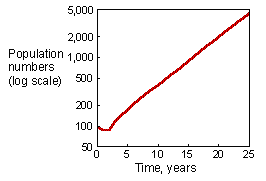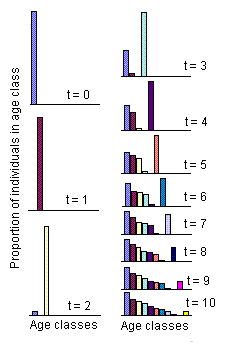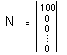7.2. Model Behavior

Two major characteristics of the behavior of Leslie model:
• A few damping oscillations are followed by an exponential growth
• Age distribution approaches a stable age distribution
These 2 features can be seen in the graphs that show simulations of sheep population dynamics:In this simulation we started from a population of 100 new-born sheep:The first graph shows exponential population growth (it becomes linear in a log scale) after several initial years. The second graph shows convergence of age distribution to a stable age distribution.

Here is an example of the model of Leslie implemented using Excel spreadsheet:

You can play with this model by changing model parameters (the matrix) and initial age-distribution.

You can simplify the analysis of matrix models using PopTools, which is a free Excel plugin developed by Greg Hood, CSIRO, Canberra, Australia.

Alexei Sharov 1/12/96